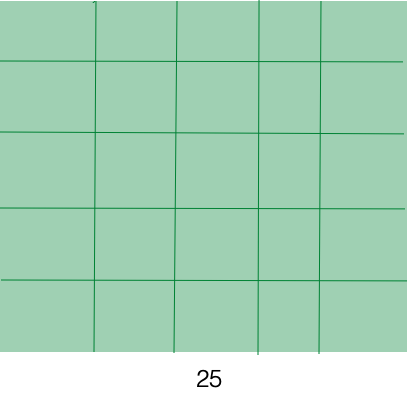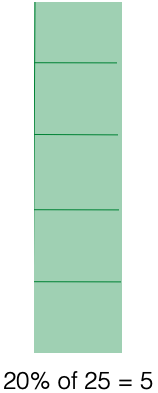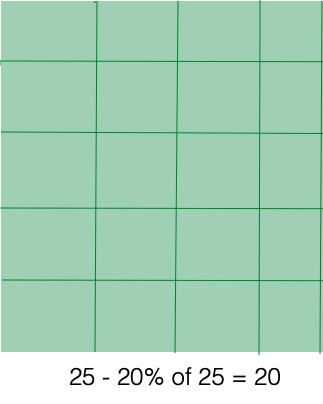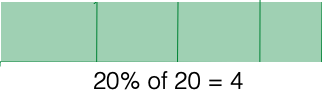## Discounts and Price IncreasesSay you want to buy a jacket. You find one online that you like for $25, but by the time you make up your mind the price has gone up 20%. You aren’t ready to pay that much, so you hesitate, and while you wait the jacket goes on sale for 20% off. How much do you have to pay for it (before tax)? Well, the original price was$25, and then there was a price increase, and then an equal price decrease, so the final price must be $25. Right? Let’s see. The price increase is 20% of$25. That’s $5. So the increased price is$30. Then the sale takes the price down 20% of $30. That’s$6. So the final price is $30 –$6 = $24.So no, the final price is not the same as the original price. I fibbed when I said the price decrease is equal to the increase. The decrease is more. Why? Because the decrease is the same percent — 20% — of a larger number. The increase is 20% of$25 and the decrease is 20% of $30.What if the sale comes first and then the price increase? Let’s see. The price decrease is 20% of$25. That’s $5. So the sale price is$20. Then inflation (or whatever) takes the price up 20% of $20. That’s$4. So the final price is $20 +$4 = $24. Same result.Same final price. Is that a fluke, or does it never make a difference whether you increase or decrease first?Say a price of$25 increases by some percentage p. To find the increased price, multiply the original price by . So in this case the new price is Now that price decreases by percentage p, so you multiply by and the final price is

On the other hand, if the decrease comes first, the final price is

The only difference is that the terms get multiplied in a different sequence. But in multiplication, changing the sequence does not change the result.

Bottom line: If a percentage increase is followed by the same percentage decrease, the final amount will not equal the original amount — but it will equal what you get if you switch the order and do the decrease first.